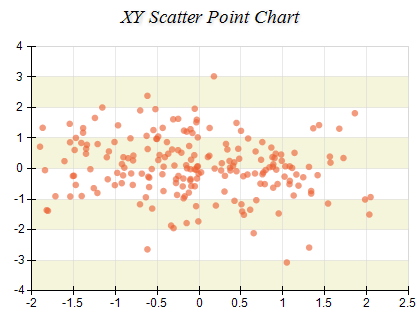﻿ Point Series
Nevron Open Vision Documentation
Chart / Charts / Types / Cartesian / Series / Point Series
In This Topic
Point Series
In This Topic

Point Charts display one or two dimensional data as points in a Cartesian coordinate system. Values are represented by the position of the points on the chart. The following image shows an xy scatter point chart:Creating a Point Series

Point series are represented by the NPointSeries type. An instance of this type must be added to the series collection of a Cartesian chart:

C#
Copy Code

NPointSeries point = new NPointSeries();

Passing Data

Point series accept data points of type NPointDataPoint. The following code snippet shows how to add data to a point series:

C#
Copy Code

// enable x values
point.UseXValues = true;

NPointDataPoint pointDataPoint = new NPointDataPoint();
pointDataPoint.Value = 10;
pointDataPoint.X = 10;

Controlling the Points Shape

The point series allows you to modify the point shape of all the data points in the series as well as individual data point. The following code snippet shows how to create a point series where all points use ellipse shape except the first one which uses star:

C#
Copy Code

NPointSeries point = new NPointSeries();

// disable data labels

point.DataLabelStyle = new NDataLabelStyle(false);

// enable x values

point.UseXValues = true;

// change the default shape to ellipse

point.Shape = ENPointShape.Ellipse;

NPointDataPoint dataPoint0 = new NPointDataPoint(0, 0);

dataPoint0.Shape = ENPointShape.Rectangle;